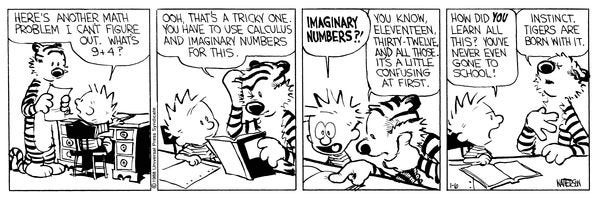## Prior Teaching

Email me if you're curious about class materials, especially if I was the instructor.

• Spring, 2021; I did not teach but was part of the Directed Reading Program; here is a survey of what my mentee learned.
• Fall, 2020; MAT 203: Calculus III with Applications
• I was on a research grant for Spring 2020 and did not teach.
• Fall, 2019; MAT 122: An Overview of Calculus with Applications and MAT 123: Precalculus
• Summer 2019; MAT 312: Applied Algebra (instructor)
• Spring 2019; MAT 123: Precalculus
• Fall 2018; MAT 131 : Calculus 1
• Summer 2018; MAT 118: Mathematical Thinking (instructor)
• Spring 2018; MAT 123: Precalculus
• Fall 2017; MAT 310: Linear Algebra

I have also been an instructor for I-STEM at Stony Brook University where I've introduced high school students to complex numbers, group theory, and the pigeonhole principle.

Here is a note on what the dx means when integrating in a Calculus I, II, or III course.

## Mathematics and Teaching Mathematics

Bill Thurston's "On Proof and Progress in Mathematics" and Paul Lockhart's "A Mathematicians Lament" summarize my main opinions concerning math and the current state of math education.

Here is my high school history teacher, Russell Brown, giving a TED talk on the current state of general education in the US. He taught me far more than just history and I am very grateful to him.

As is often the case, "Calvin and Hobbes" speaks to the truth of the matter (all credit to Bill Watterson).Many of my students are not so unlike Calvin who concludes that 3 + 8 = 6. If they ask themselves the simple question of, "If I start off with 8 and I add something positive to it like 3, could I end with something smaller than 8?", they'll find that 6 cannot be the correct answer. Assuming they know 6 is smaller than 8. But unfortunately, it seems American public schools do not teach students to ask such questions. In fact, it doesn't seem to teach students to ask any sort of meaningful question. Questions that my calculus students voluntarily ask are usually along the lines of, "Why does x/(1/2) = 2x?" There is also the classic question which high school students often ask but college students no longer care to ask: "When will we use this in life?" The answer is, "I don't know because I don't know what your hopes and dreams are for a career. You probably don't know all the kinds of career paths out there." However, some teachers fall into the trap of answering the question which, most of the time, the student wasn't actually seeking. The question is really a statement: "I do not feel motivated to learn this." And who can blame them when the way math is taught in the US is akin to a piano teacher having a student only play the C major scale for weeks on end with nothing to look forward to except for the G major scale? (an example of Jacob Lurie).

Unfortunately, I don't think there is much that can be done once a student is at the college level. It's not impossible but typically, either they already like math or their interest in math has long been buried, the grave long gone cold. The solution needs to begin much earlier, at the primary school level. My hope is like that of Paul Lockhart: let kids explore math in a way that feels like discovery. Forget about the supposedly "novel" ways of multiplying numbers by first drawing boxes with diagonals. Tell stories instead of definitions and then ask the students offer their own definitions to compare to the actual one. Make critical thinking fun. Teach them to ask meaningful questions.Here is another instructive lesson. When desperate, students will often make up imaginative answers and rules that are nonsensical. For example, when asked to find the height of a building in a word problem, is is not so uncommon for students to give a negative answer. Or they will say that (x+y)^2 = x^2 + y^2 over rings where the characteristic is not 2. Which is plainly false if we just try an example with x = +1 and y = -1. Then (x+y)^2 = 0 while x^2 + y^2 = 2; they don't equal. These are two ideas that schools seem to fail at teaching: checking to see if an answer makes sense and testing a claim on some simple cases.

I think this is an area which we have some hope of improving at the college level. But one needs to communicate to students that math isn't just about getting an answer but about interpreting it. It isn't just about trying to be right because what's the use of that if you don't even know why something is correct? It's not just about what is right but also seeing why something is wrong. And for the sake of Pete, math is not about arguing for a few extra points on an exam to grovel through Calculus I and into Calculus II where you still don't know what the graph of y=e^x looks like.